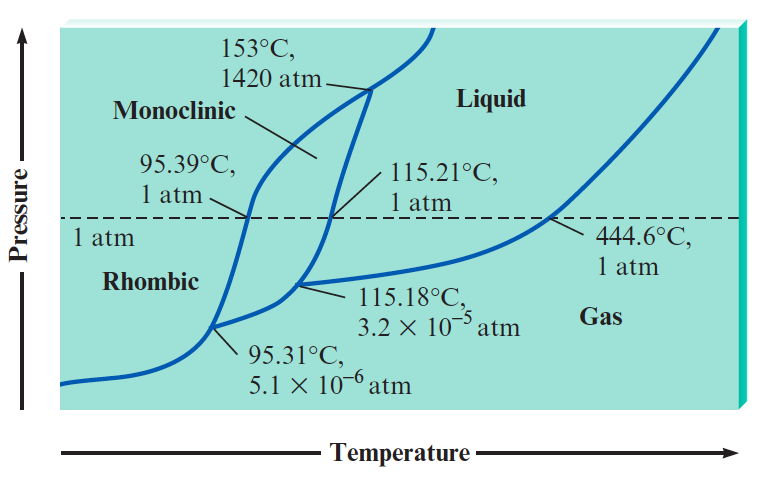# Problem: Sulfur exhibits two solid phases, rhombic and monoclinic. Use the accompanying phase diagram for sulfur to answer the following questions. (The phase diagram is not to scale.)Which is the densest phase?

⚠️Our tutors found the solution shown to be helpful for the problem you're searching for. We don't have the exact solution yet.

###### Problem Details

Sulfur exhibits two solid phases, rhombic and monoclinic. Use the accompanying phase diagram for sulfur to answer the following questions. (The phase diagram is not to scale.)Which is the densest phase?